# How to Calculate and Solve for Rated Static Head | Design of Gating System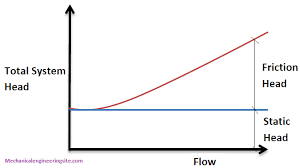The image above represents rated static head.

To compute for rated static head, three essential parameters are needed and these parameters are Height of the Sprue from its Top to the Point of Metal Entry into the Mould Cavity (H), Height of the Casting System from its top (P) and Height of the Casting (C).

The formula for calculating rated static head:

Hs = H – P2 / 2C

Where:

H = Height of the Sprue from its Top to the Point of Metal Entry into the Mould Cavity
P = Height of the Casting from its Top
C = Height of the Casting

Let’s solve an example;
Find the rated static head when the height of the sprue from its top to the point of metal entry into the mould cavity is 9,the height of the casting from its top is 5 and the height of the casting is 11.

This implies that;

H = Height of the Sprue from its Top to the Point of Metal Entry into the Mould Cavity = 9
P = Height of the Casting from its Top = 5
C = Height of the Casting = 11

Hs = H – P2 / 2C
Hs = 9 – 52 / 2 x 11
Hs = 9 – 25 / 22
Hs = 9 – 1.136
Hs = 7.86

Therefore, the rated static head is 7.86 m.

Calculating the Height of the Sprue from its Top to the Point of Metal Entry into the Mould Cavity when the Rated Static Head, Height of the Casting System from its Top and the Height of the Casting is Given.

H = HsP2 / 2C

Where;

H = Height of the Sprue from its Top to the Point of Metal Entry into the Mould Cavity
P = Height of the Casting from its Top
C = Height of the Casting

Let’s solve an example;
Find the height of the sprue when the rated static head is 25, the height of the casting system from its top is 8 and the height of the casting is 4.

This implies that;

Hs = Rated Static Head = 25
P = Height of the Casting from its Top = 8
C = Height of the Casting = 4

H = HsP2 / 2C
H = 25 + 82 / 2 x 4
H = 25 + 64 / 8
H = 25 + 8
H = 33

Therefore, the height of the casting system from its top is 33.

Calculating the Height of the Casting System from its Top when the Rated Static Head, the Height of the Sprue from its Top to the Point of Metal Entry into Mould Cavity and the Height of the Casting is Given.

p = – √(Hs – H) x 2c

Where;

P = Height of the Casting from its Top
H = Height of the Sprue from its Top to the Point of Metal Entry into the Mould Cavity
C = Height of the Casting

Let’s solve an example;
Find the height of the casting from its top when the rated static head is 34, the height of the sprue from its top to the point of metal entry into the mould cavity is 28 and the height of the casting is 14.

This implies that;

Hs = Rated Static Head = 34
H = Height of the Sprue from its Top to the Point of Metal Entry into the Mould Cavity = 28
C = Height of the Casting = 14

p = – √(Hs – H) x 2c
p = – √(34 – 28) x 2(14)
p = – √6 x 28
p = – √168
p = – 12.96

Therefore, the height of the casting system from its top is – 12.96.

Calculating the Height of the Casting when the Rated Static Head, the Height of the Sprue from its Top to the Point of Metal Entry into Mould Cavity and the Height of the casting system from its top is Given.

c = – p2 / (Hs – H) x 2

Where;

C = Height of the Casting
H = Height of the Sprue from its Top to the Point of Metal Entry into the Mould Cavity
P = Height of the Casting from its Top

Let’s solve an example;
Find the height of the casting when the rated static head is 24, the height of the sprue from its top to the point of metal entry into the mould cavity is 12 and the height of the casting system from its top is 10.

This implies that;

Hs = Rated Static Head = 24
H = Height of the Sprue from its Top to the Point of Metal Entry into the Mould Cavity = 12
P = Height of the Casting from its Top = 10

c = – p2 / (Hs – H) x 2
c = – 102 / (24 – 12) x 2
c = – 100 / 12 x 2
c = – 100 / 24
c = – 4.16

Therefore, the height of the casting is – 4.16.

Nickzom Calculator – The Calculator Encyclopedia is capable of calculating the rated static head.

To get the answer and workings of the rated static head using the Nickzom Calculator – The Calculator Encyclopedia. First, you need to obtain the app.

You can get this app via any of these means:

To get access to the professional version via web, you need to register and subscribe for NGN 1,500 per annum to have utter access to all functionalities.
You can also try the demo version via https://www.nickzom.org/calculator

Apple (Paid) – https://itunes.apple.com/us/app/nickzom-calculator/id1331162702?mt=8
Once, you have obtained the calculator encyclopedia app, proceed to the Calculator Map, then click on Materials & Metallurgical under Engineering.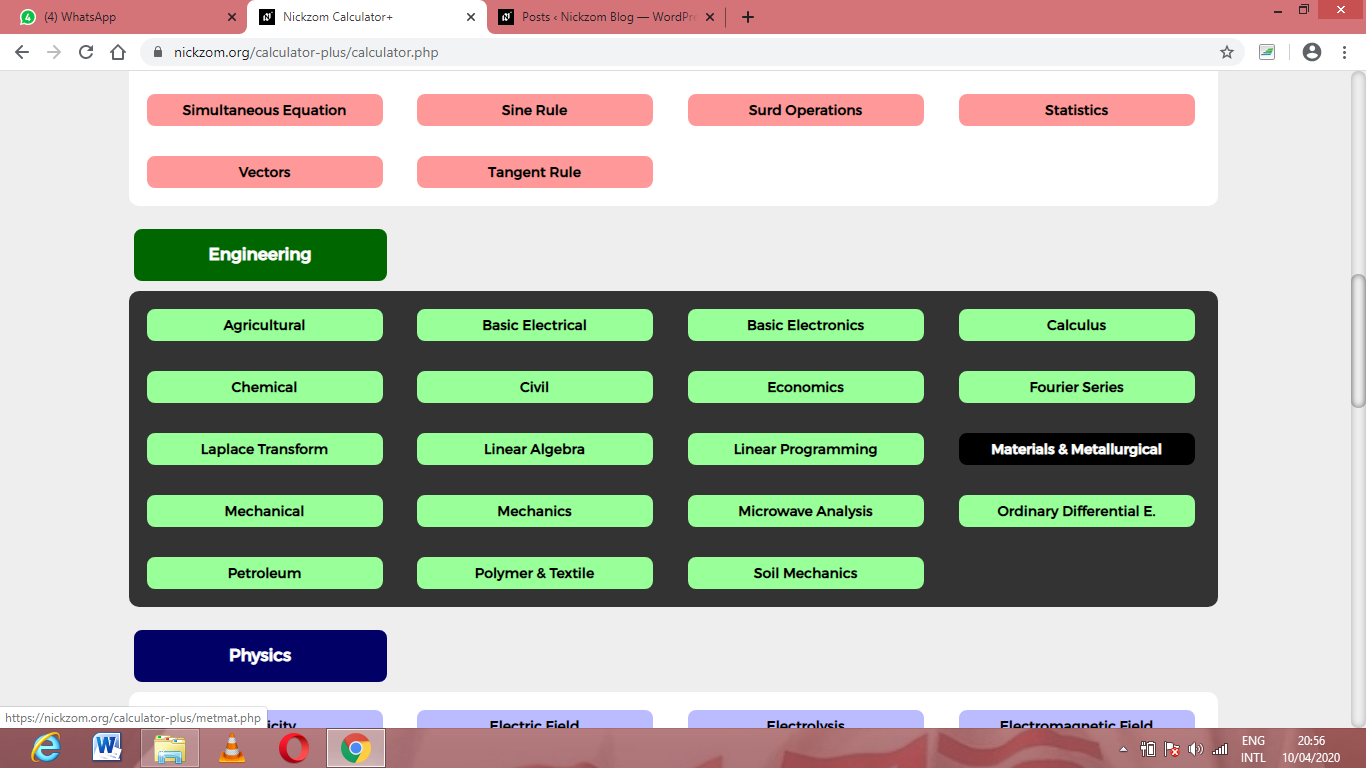Now, Click on Foundry Technology under Material & MetallurgicalNow, Click on Design of Gating System under Foundry Technology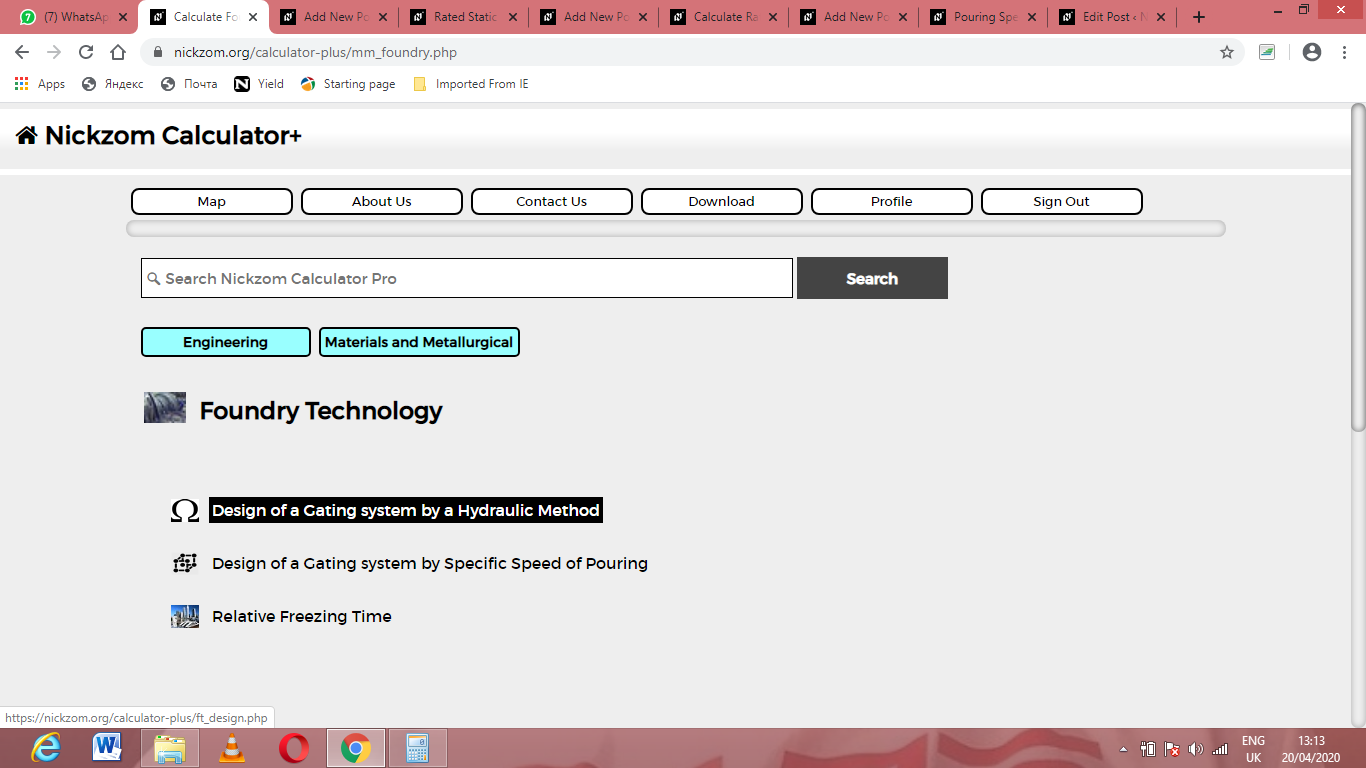Now, Click on Rated Static Head under Design of Gating System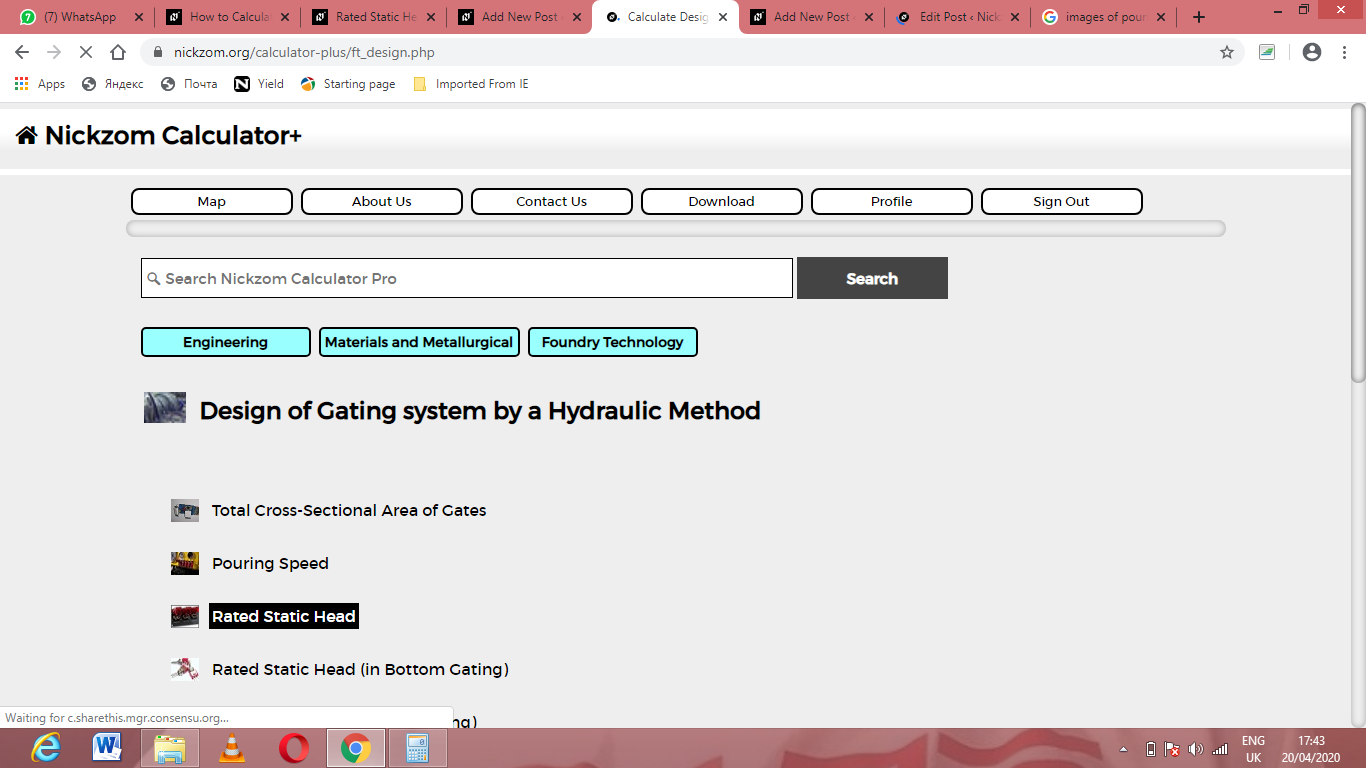The screenshot below displays the page or activity to enter your values, to get the answer for the rated static head according to the respective parameters which are the Height of the Sprue from its Top to the Point of Metal Entry into the Mould Cavity (H), Height of the Casting System from its top (P) and Height of the Casting (C).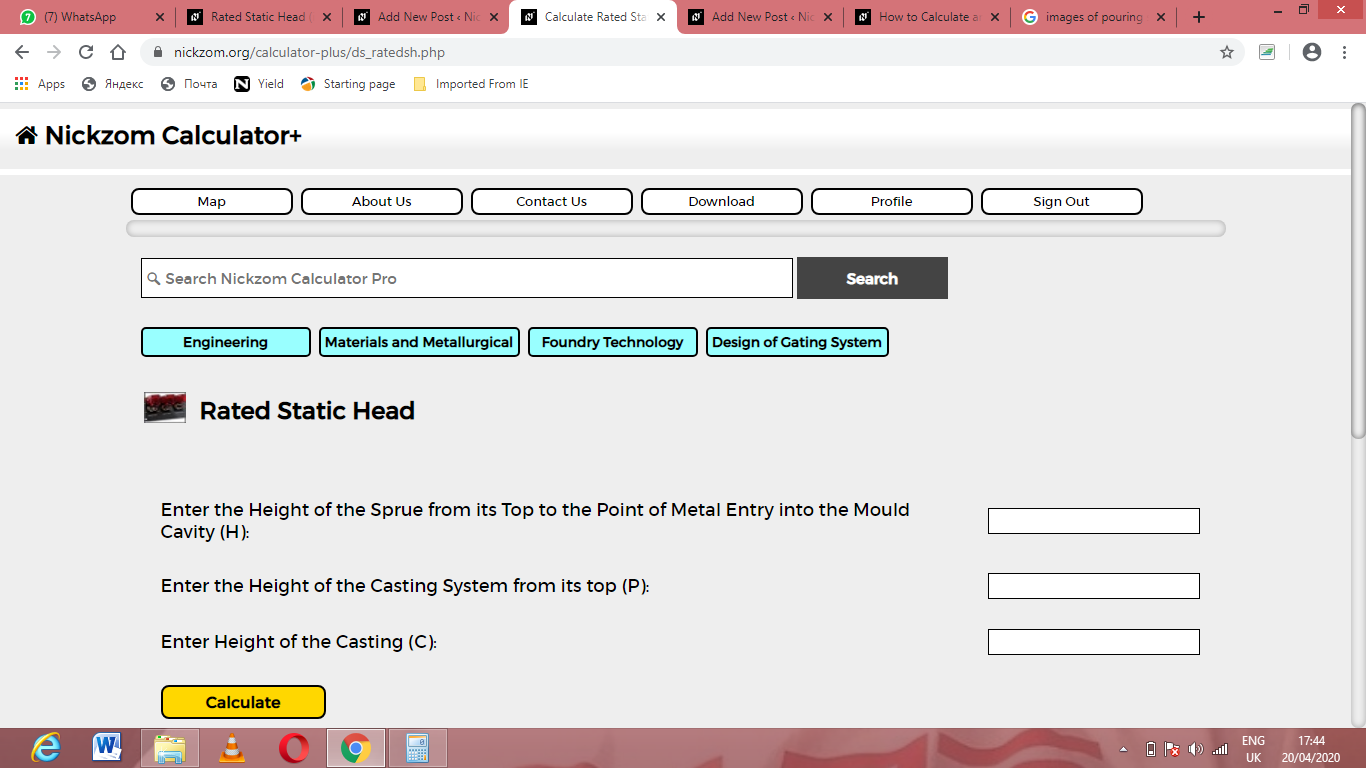Now, enter the values appropriately and accordingly for the parameters as required by the Height of the Sprue from its Top to the Point of Metal Entry into the Mould Cavity (H) is 9, Height of the Casting System from its top (P) is 5 and Height of the Casting (C) is 11.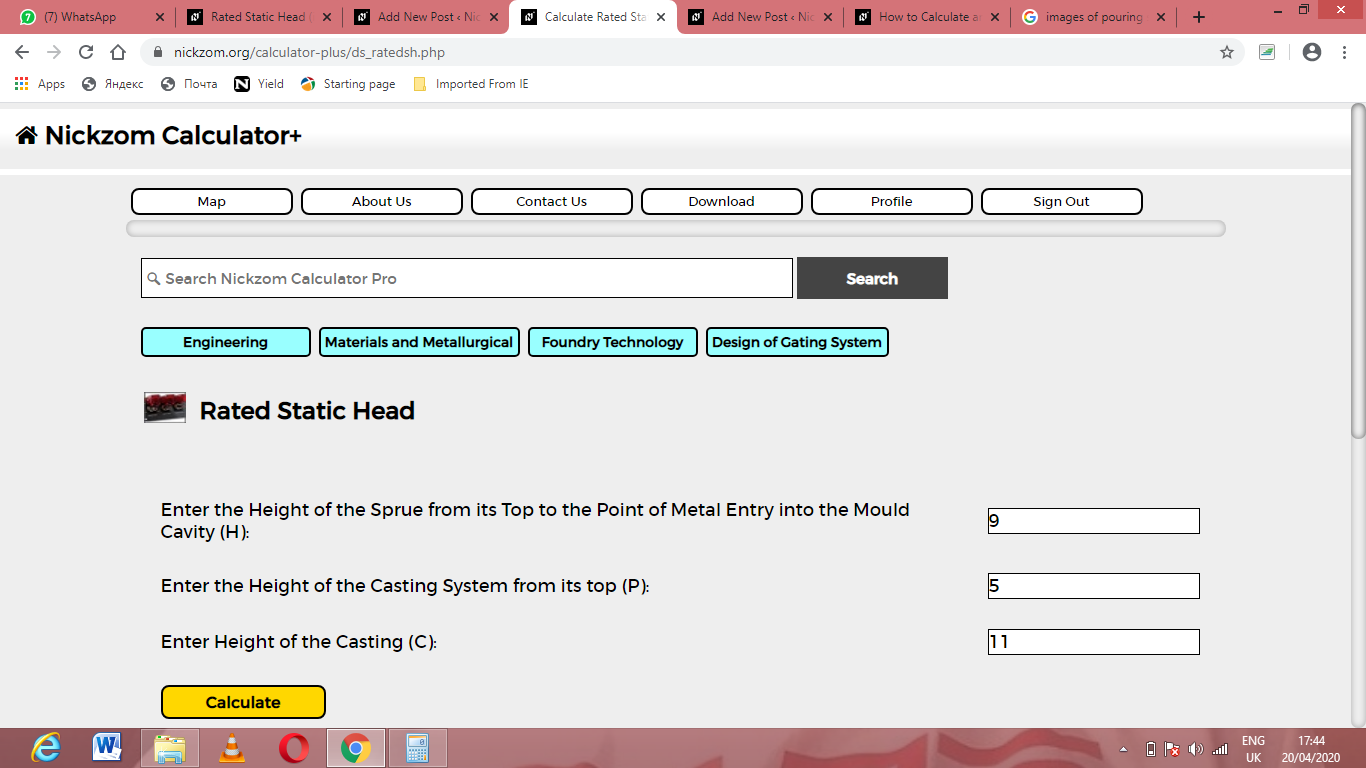Finally, Click on Calculate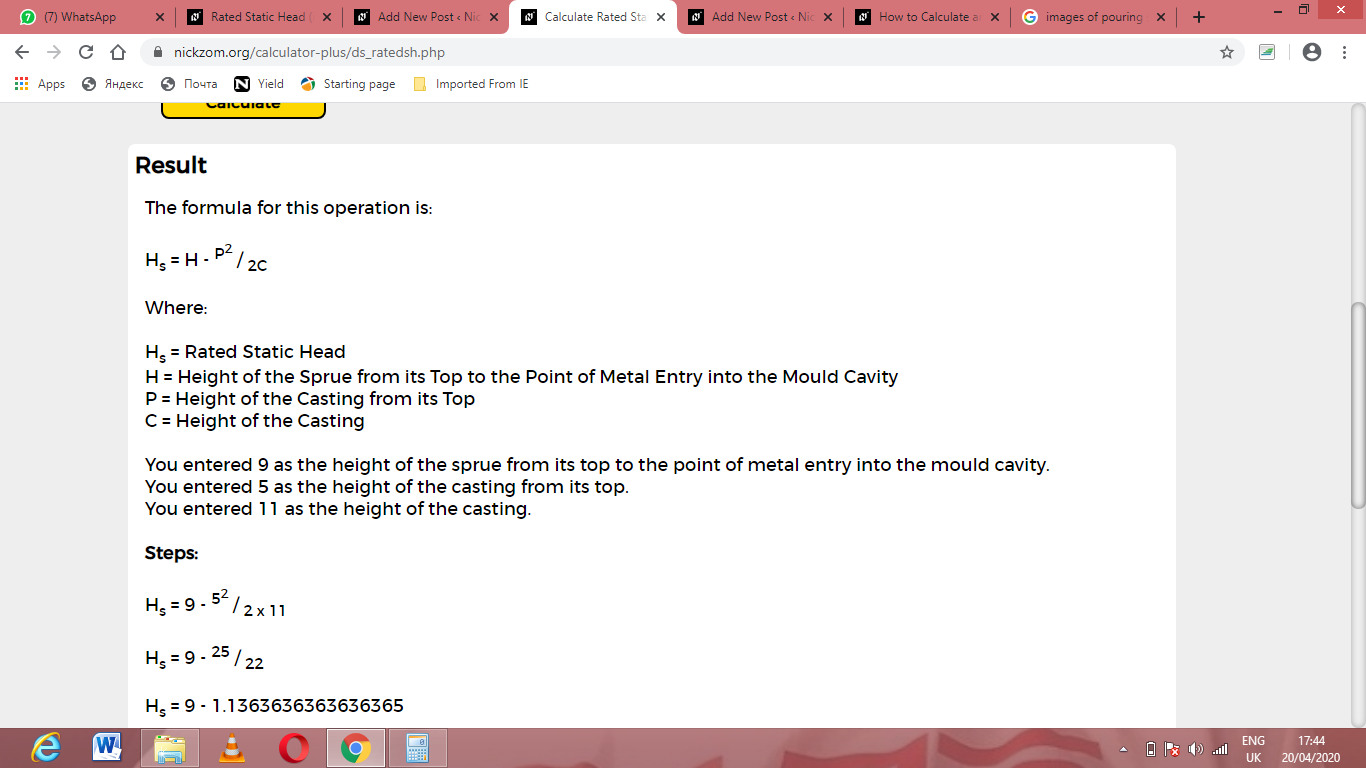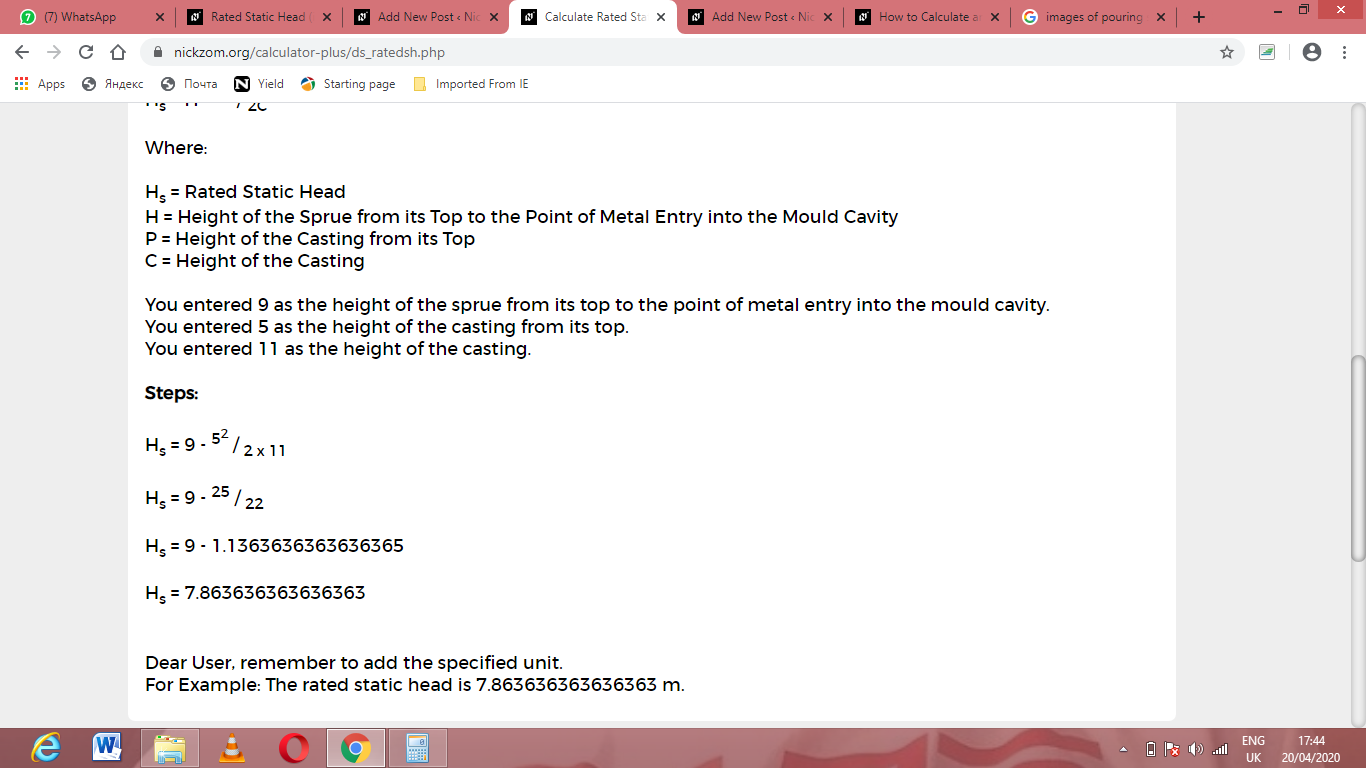As you can see from the screenshot above, Nickzom Calculator– The Calculator Encyclopedia solves for the rated static head and presents the formula, workings and steps too.